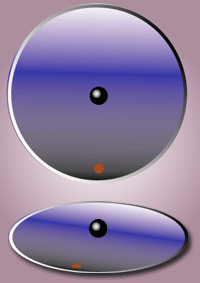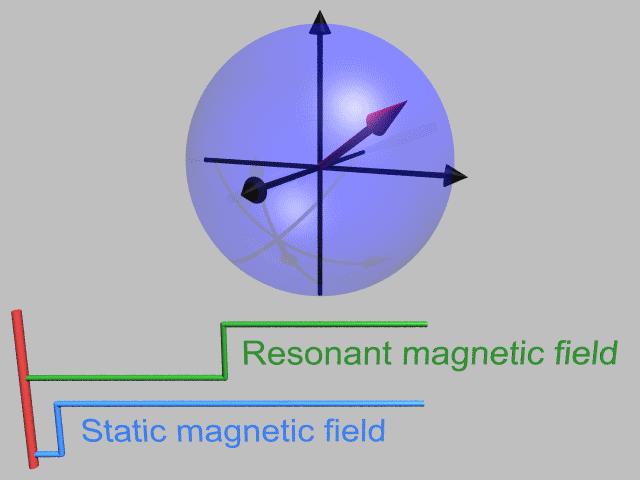# Rotating reference frame

A rotating frame of reference is a special case of a non-inertial reference frame that is rotating relative to an inertial reference frame. An everyday example of a rotating reference frame is the surface of the Earth. (This article considers only frames rotating about a fixed axis. For more general rotations, see Euler angles.)

## Fictitious forces

Main article: Fictitious forces

All non-inertial reference frames exhibit fictitious forces. Rotating reference frames are characterized by three fictitious forces:

and, for non-uniformly rotating reference frames,

Scientists living in a rotating box can measure the speed and direction of their rotation by measuring these fictitious forces. For example, Léon Foucault was able to show the Coriolis force that results from the Earth's rotation using the Foucault pendulum. If the Earth were to rotate many times faster, these fictitious forces could be felt by humans, as they are when on a spinning carousel.

## Relating rotating frames to stationary frames

The following is a derivation of the formulas for accelerations as well as fictitious forces in a rotating frame. It begins with the relation between a particle's coordinates in a rotating frame and its coordinates in an inertial (stationary) frame. Then, by taking time derivatives, formulas are derived that relate the velocity of the particle as seen in the two frames, and the acceleration relative to each frame. Using these accelerations, the fictitious forces are identified by comparing Newton's second law as formulated in the two different frames.

### Relation between positions in the two frames

To derive these fictitious forces, it's helpful to be able to convert between the coordinatesof the rotating reference frame and the coordinatesof an inertial reference frame with the same origin. If the rotation is about theaxis with a constant angular velocity, or, and the two reference frames coincide at time, the transformation from rotating coordinates to inertial coordinates can be writtenwhereas the reverse transformation isThis result can be obtained from a rotation matrix.

Introduce the unit vectorsrepresenting standard unit basis vectors in the rotating frame. The time-derivatives of these unit vectors are found next. Suppose the frames are aligned at t = 0 and the z-axis is the axis of rotation. Then for a counterclockwise rotation through angle Ωt:where the (x, y) components are expressed in the stationary frame. Likewise,Thus the time derivative of these vectors, which rotate without changing magnitude, iswhere. This result is the same as found using a vector cross product with the rotation vectorpointed along the z-axis of rotation, namely,whereis eitheror.

### Time derivatives in the two frames

Introduce the unit vectorsrepresenting standard unit basis vectors in the rotating frame. As they rotate they will remain normalized. If we let them rotate at the speed ofabout an axisthen each unit vectorof the rotating coordinate system abides by the following equation:Then if we have a vector function,and we want to examine its first derivative we have (using the product rule of differentiation):whereis the rate of change ofas observed in the rotating coordinate system. As a shorthand the differentiation is expressed as:This result is also known as the Transport Theorem in analytical dynamics and is also sometimes referred to as the Basic Kinematic Equation.

### Relation between velocities in the two frames

A velocity of an object is the time-derivative of the object's position, orThe time derivative of a positionin a rotating reference frame has two components, one from the explicit time dependence due to motion of the particle itself, and another from the frame's own rotation. Applying the result of the previous subsection to the displacement, the velocities in the two reference frames are related by the equationwhere subscript i means the inertial frame of reference, and r means the rotating frame of reference.

### Relation between accelerations in the two frames

Acceleration is the second time derivative of position, or the first time derivative of velocitywhere subscript i means the inertial frame of reference. Carrying out the differentiations and re-arranging some terms yields the acceleration in the rotating reference framewhereis the apparent acceleration in the rotating reference frame, the termrepresents centrifugal acceleration, and the termis the coriolis acceleration.

### Newton's second law in the two frames

When the expression for acceleration is multiplied by the mass of the particle, the three extra terms on the right-hand side result in fictitious forces in the rotating reference frame, that is, apparent forces that result from being in a non-inertial reference frame, rather than from any physical interaction between bodies.

Using Newton's second law of motion, we obtain:whereis the mass of the object being acted upon by these fictitious forces. Notice that all three forces vanish when the frame is not rotating, that is, whenFor completeness, the inertial accelerationdue to impressed external forcescan be determined from the total physical force in the inertial (non-rotating) frame (for example, force from physical interactions such as electromagnetic forces) using Newton's second law in the inertial frame:Newton's law in the rotating frame then becomesIn other words, to handle the laws of motion in a rotating reference frame:

Treat the fictitious forces like real forces, and pretend you are in an inertial frame.
Louis N. Hand, Janet D. Finch Analytical Mechanics, p. 267
Obviously, a rotating frame of reference is a case of a non-inertial frame. Thus the particle in addition to the real force is acted upon by a fictitious force...The particle will move according to Newton's second law of motion if the total force acting on it is taken as the sum of the real and fictitious forces.
HS Hans & SP Pui: Mechanics; p. 341
This equation has exactly the form of Newton's second law, except that in addition to F, the sum of all forces identified in the inertial frame, there is an extra term on the right...This means we can continue to use Newton's second law in the noninertial frame provided we agree that in the noninertial frame we must add an extra force-like term, often called the inertial force.
John R. Taylor: Classical Mechanics; p. 328

## Centrifugal force

In classical mechanics, centrifugal force is an outward force associated with rotation. Centrifugal force is one of several so-called pseudo-forces (also known as inertial forces), so named because, unlike real forces, they do not originate in interactions with other bodies situated in the environment of the particle upon which they act. Instead, centrifugal force originates in the rotation of the frame of reference within which observations are made.

## Coriolis effect

Main article: Coriolis effectThe mathematical expression for the Coriolis force appeared in an 1835 paper by a French scientist Gaspard-Gustave Coriolis in connection with hydrodynamics, and also in the tidal equations of Pierre-Simon Laplace in 1778. Early in the 20th century, the term Coriolis force began to be used in connection with meteorology.

Perhaps the most commonly encountered rotating reference frame is the Earth. Moving objects on the surface of the Earth experience a Coriolis force, and appear to veer to the right in the northern hemisphere, and to the left in the southern. Movements of air in the atmosphere and water in the ocean are notable examples of this behavior: rather than flowing directly from areas of high pressure to low pressure, as they would on a non-rotating planet, winds and currents tend to flow to the right of this direction north of the equator, and to the left of this direction south of the equator. This effect is responsible for the rotation of large cyclones (see Coriolis effects in meteorology).

## Euler force

Main article: Euler force

In classical mechanics, the Euler acceleration (named for Leonhard Euler), also known as azimuthal acceleration or transverse acceleration is an acceleration that appears when a non-uniformly rotating reference frame is used for analysis of motion and there is variation in the angular velocity of the reference frame's axis. This article is restricted to a frame of reference that rotates about a fixed axis.

The Euler force is a fictitious force on a body that is related to the Euler acceleration by F  = ma, where a is the Euler acceleration and m is the mass of the body.

## Use in magnetic resonance

It is convenient to consider magnetic resonance in a frame that rotates at the Larmor frequency of the spins. This is illustrated in the animation below. The rotating wave approximation may also be used.## References

1. Vladimir Igorević Arnolʹd (1989). Mathematical Methods of Classical Mechanics (2nd ed.). Springer. p. 130. ISBN 978-0-387-96890-2.
2. Cornelius Lanczos (1986). The Variational Principles of Mechanics (Reprint of Fourth Edition of 1970 ed.). Dover Publications. Chapter 4, §5. ISBN 0-486-65067-7.
3. John R Taylor (2005). Classical Mechanics. University Science Books. p. 342. ISBN 1-891389-22-X.
4. Corless, Martin. "Kinematics" (PDF). Aeromechanics I Course Notes. Purdue University. p. 213. Retrieved 18 July 2011.
5. LD Landau & LM Lifshitz (1976). Mechanics (Third ed.). p. 128. ISBN 978-0-7506-2896-9.
6. Louis N. Hand; Janet D. Finch (1998). Analytical Mechanics. Cambridge University Press. p. 267. ISBN 0-521-57572-9.
7. HS Hans & SP Pui (2003). Mechanics. Tata McGraw-Hill. p. 341. ISBN 0-07-047360-9.
8. John R Taylor (2005). Classical Mechanics. University Science Books. p. 328. ISBN 1-891389-22-X.
9. Robert Resnick & David Halliday (1966). Physics. Wiley. p. 121. ISBN 0-471-34524-5.
10. Jerrold E. Marsden; Tudor S. Ratiu (1999). Introduction to Mechanics and Symmetry: A Basic Exposition of Classical Mechanical Systems. Springer. p. 251. ISBN 0-387-98643-X.
11. John Robert Taylor (2005). Classical Mechanics. University Science Books. p. 343. ISBN 1-891389-22-X.
12. Stephen T. Thornton & Jerry B. Marion (2004). Classical Dynamics of Particles and Systems (5th ed.). Belmont CA: Brook/Cole. Chapter 10. ISBN 0-534-40896-6.
13. David McNaughton. "Centrifugal and Coriolis Effects". Retrieved 2008-05-18.
14. David P. Stern. "Frames of reference: The centrifugal force". Retrieved 2008-10-26.
15. David Morin (2008). Introduction to classical mechanics: with problems and solutions. Cambridge University Press. p. 469. ISBN 0-521-87622-2.
16. Grant R. Fowles & George L. Cassiday (1999). Analytical Mechanics (6th ed.). Harcourt College Publishers. p. 178.
17. Richard H Battin (1999). An introduction to the mathematics and methods of astrodynamics. Reston, VA: American Institute of Aeronautics and Astronautics. p. 102. ISBN 1-56347-342-9.
18. Jerrold E. Marsden; Tudor S. Ratiu (1999). Introduction to Mechanics and Symmetry: A Basic Exposition of Classical Mechanical Systems. Springer. p. 251. ISBN 0-387-98643-X.